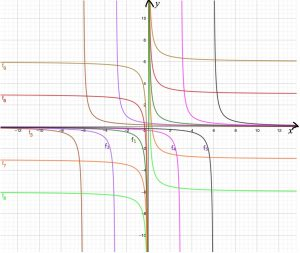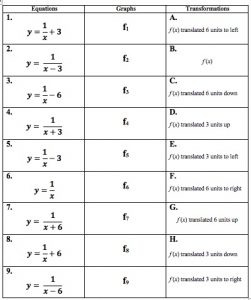1. Which one of the graphs represents the function   $f(x) = \frac{1}{x}$?

The table below has been jumbled up. Match the graphs labelled f1 to f9 to their equations in the table below and to the descriptions of the transformations of $f(x) = \frac{1}{x}$Download the worksheet, cut up a copy of the table and rearrange the pieces into 9 matching sets.

Only one branch of the graphs are labelled in the diagram above. Label the other branch of each graph.

2.  Given: $g(x)=\frac{3}{x+1}-2$

2.1  Write down the coordinates of the  x-intercept of g.

2.2  Write down the coordinates of the y-intercept of g.

2.3  Write down the equation of the vertical asymptote of g.

2.4   Write down the equation of the horizontal asymptote of g.

2.5   Sketch the graph of g.

Tagged with: